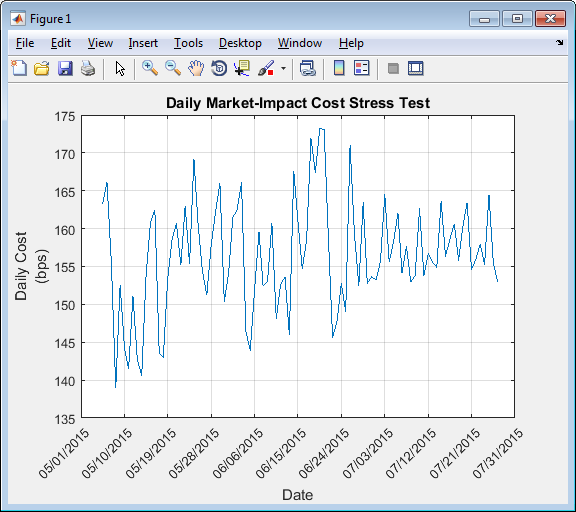## Conduct Stress Test on Portfolio

This example shows how to conduct a stress test on a set of stocks using transaction cost analysis from the Kissell Research Group.

• Estimate historical market-impact costs and the corresponding dollar values for the specified date range.

• Use trading costs to screen stocks in a portfolio and estimate the cost to liquidate or purchase a specified number of shares.

• Analyze trading costs during volatile periods of time such as a financial crisis, flash crash, or debt crisis.

To access the example code, enter ```edit KRGStressTestingExample.m``` at the command line.

### Retrieve Market-Impact Parameters and Load Historical Data

Retrieve the market-impact data from the Kissell Research Group FTP site. Connect to the FTP site using the `ftp` function with a user name and password. Navigate to the `MI_Parameters` folder and retrieve the market-impact data in the `MI_Encrypted_Parameters.csv` file. `miData` contains the encrypted market-impact date, code, and parameters.

```f = ftp('ftp.kissellresearch.com','username','pwd'); mget(f,'MI_Encrypted_Parameters.csv'); close(f) miData = readtable('MI_Encrypted_Parameters.csv','delimiter', ... ',','ReadRowNames',false,'ReadVariableNames',true);```

Load the example data `TradeDataStressTest` from the file `KRGExampleData.mat`, which is included with the Datafeed Toolbox™.

`load KRGExampleData TradeDataStressTest`

For a description of the example data, see Kissell Research Group Data Sets.

Create a Kissell Research Group transaction cost analysis object `k`. Specify initial settings for the date, market-impact code, and number of trading days.

`k = krg(miData,datetime('today'),1,250);`

### Prepare Data for Stress Testing

Specify the date range from May 1, 2015, through July 31, 2015.

```startDate = '5/1/2015'; endDate = '7/31/2015'; ```

Determine the number of stocks `numStocks` in the portfolio. Create a date range `dateRange` from the specified dates. Find the number of days `numDates` in the date range.

```numStocks = length(TradeDataStressTest.Symbol); dateRange = (datenum(startDate):datenum(endDate))'; numDates = length(dateRange); ```

Preallocate the output data table `o`.

```outLength = numStocks*numDates; symbols = TradeDataStressTest.Symbol(:,ones(1,numDates)); sides = TradeDataStressTest.Side(:,ones(1,numDates)); dates = dateRange(:,ones(1,numStocks))'; o = table(symbols(:),sides(:),dates(:),NaN(outLength,1),NaN(outLength,1), ... 'VariableNames',{'Symbol','Side','Date','MI','MIDollar'}); ```

Ensure that the number of shares is a positive value using the `abs` function.

`TradeDataStressTest.Shares = abs(TradeDataStressTest.Shares);`

```TradeDataStressTest.TradeTime = TradeDataStressTest.TradeTime ... .* TradeDataStressTest.ADV; TradeDataStressTest.POV = krg.tradetime2pov(TradeDataStressTest.TradeTime, ... TradeDataStressTest.Shares); ```

### Conduct Stress Test by Estimating Historical Market-Impact Costs

Estimate the historical market-impact costs for each stock in the portfolio for the date range using `marketImpact`. Convert market-impact cost from decimal into local dollars. Retrieve the resulting data in the output data table `o`.

```kk = 1; for ii = dateRange(1):dateRange(end) for jj = 1:numStocks k.MiCode = TradeDataStressTest.MICode(jj); k.MiDate = ii; o.MI(kk) = marketImpact(k,TradeDataStressTest(jj,:)); o.MIDollar(kk) = (TradeDataStressTest.Shares(jj) ... * TradeDataStressTest.Price(jj)) ... * o.MI(kk) /10000 * TradeDataStressTest.FXRate(jj); kk = kk + 1; end end```

Display the first three rows of output data.

`o(1:3,:)`
```ans = Symbol Side Date MI MIDollar ______ ____ _________ _____ ________ 'A' 1.00 736085.00 3.84 384.31 'B' 1.00 736085.00 11.43 14292.24 'C' 1.00 736085.00 32.69 20430.65```

The output data contains these variables:

• Stock symbol

• Side

• Historical market-impact cost in basis points

• Historical market-impact value in local dollars

Retrieve the daily market-impact cost `dailyCost`. Determine the number of days `numDays` in the output data. Loop through the data and sum the market-impact costs for individual stocks for each day.

```numDays = length(o.Date)/numStocks; idx = 1; for i = 1:numDays dailyCost.Date(i) = o.Date(idx); dailyCost.DailyMiCost(i) = sum(o.MI(idx:idx+(numStocks-1))); idx = idx+numStocks; end```

Display the daily market-impact cost in the specified date range. This figure demonstrates how market-impact costs change over time.

```plot(b.Date,b.DailyMiCost) ylabel({'Daily Cost','(bps)'}) title('Daily Market-Impact Cost Stress Test') xlabel('Date') grid on xData = linspace(b.Date(1),b.Date(92),11); a = gca; a.XAxis.TickLabels = datestr(xData,'mm/dd/yyyy'); a.XTickLabelRotation = 45; ```Kissell, Robert. “Creating Dynamic Pre-Trade Models: Beyond the Black Box.” Journal of Trading. Vol. 6, Number 4, Fall 2011, pp. 8–15.

 Kissell, Robert. “TCA in the Investment Process: An Overview.” Journal of Index Investing. Vol. 2, Number 1, Summer 2011, pp. 60–64.

 Kissell, Robert. The Science of Algorithmic Trading and Portfolio Management. Cambridge, MA: Elsevier/Academic Press, 2013.

 Chung, Grace and Robert Kissell. “An Application of Transaction Costs in the Portfolio Optimization Process.” Journal of Trading. Vol. 11, Number 2, Spring 2016, pp. 11–20.